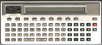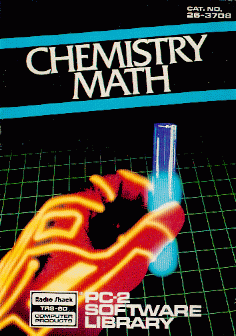##### TRS-80 PC-2 (and Sharp PC-1500) : Chemistry Math

Radio Shack TRS-80 PC-2 Chemistry Math software(*).PC-2 Chemistry Math: © 1982 Tandy Corporation. All rights reserved. The Chemistry Math™ package is a tool for students who are familiar with the fundamentals of chemistry. The first eight programs perform basic chemistry calculations. The last two programs are handy references; one converts English units of measure to metric units, one is a periodic table of the elements. "Chemistry Math" consists of: WTWTVOL - Solves weight-weight and weight-volume problems. EQUILIB - Calculates acid equilibria. IDEALGAS - Performs ideal gas calculations. MOLNORM - Calculates molarity/normality of solutions. BPFP - Calculates boiling and freezing poinds of solutions. KSP - Calculates solubility products. FORMWTS - Calculates molecular weights. PH - Calculates pH, OH-, and H+ concentrations of solutions. METRIC - Converts English units of measure to metric units. ATOMWTS - Finds atomic weights. The PC-2 displays the results of its calculations to its full available accuracy - up to 10 digits. If you wish, you can receive a printout of each problem and its answer.